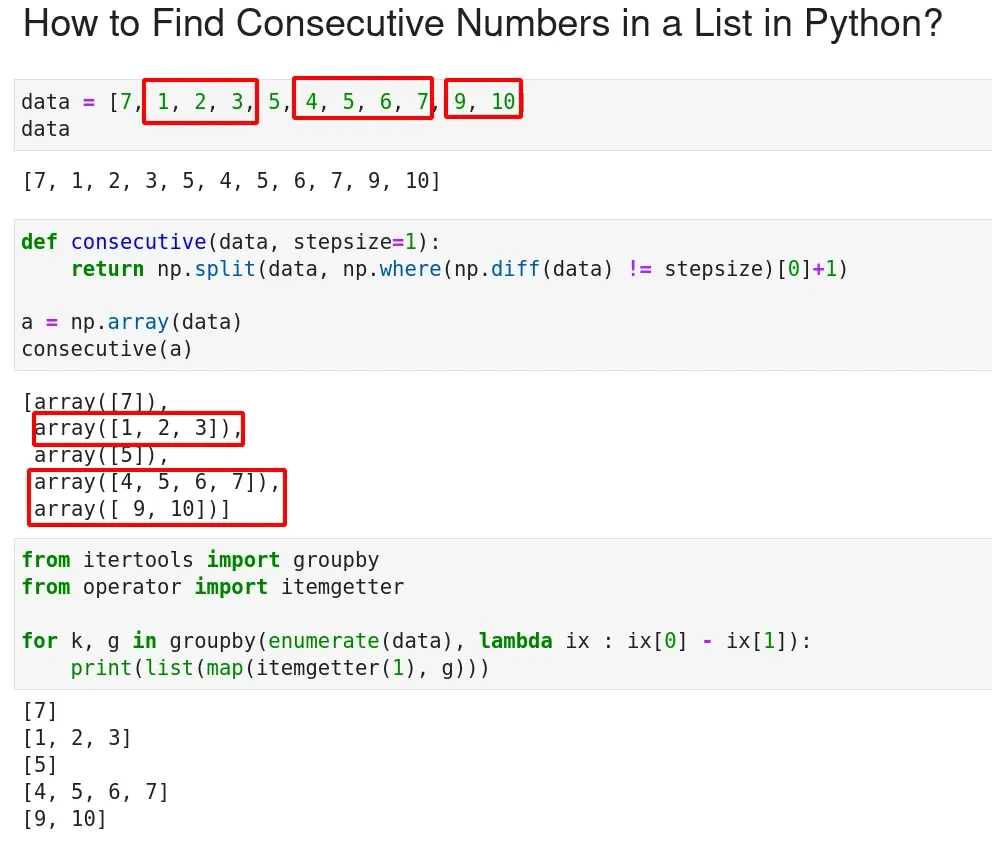To find consecutive numbers in a list in PythonWe can use different techniques. Below you can find 3 examples of grouping consecutive numbers in list/array in Python:## find consecutive numbers with numpy

My favorite solution is by module `numpy` we can detect consecutive numbers in array by:

``````def consecutive(data, stepsize=1):
return np.split(data, np.where(np.diff(data) != stepsize)+1)

a = np.array([7, 1, 2, 3, 5, 4, 5, 6, 7, 9, 10])
consecutive(a)
``````

result:

``````
[1, 2, 3]

[4, 5, 6, 7]
[9, 10]
``````

The code works as follow:

• `np.diff(data)` - get difference of items
• `array([-6, 1, 1, 2, -1, 1, 1, 1, 2, 1])`
• `np.where(np.diff(data) != 1) + 1`
• get difference if different than 1
• extract index and add 1
• array([1, 4, 5, 9])
• split the array on those indexes

## itertools + groupby to detect consecutive numbers

As an alternative we can use another solution based on `itertools` and `groupby`.

``````from itertools import groupby
from operator import itemgetter
data = [7, 1, 2, 3, 5, 4, 5, 6, 7, 9, 10]
for k, g in groupby(enumerate(data), lambda ix : ix - ix):
print(list(map(itemgetter(1), g)))
``````

result:

``````[array(),
array([1, 2, 3]),
array(),
array([4, 5, 6, 7]),
array([ 9, 10])]
``````

To find out how the code get consecutive numbers we can run this code:

``````for k, g in groupby(enumerate(data), lambda ix : ix - ix):
print(k, list(g))
``````

which give us:

``````-7 [(0, 7)]
0 [(1, 1), (2, 2), (3, 3)]
-1 [(4, 5)]
1 [(5, 4), (6, 5), (7, 6), (8, 7)]
0 [(9, 9), (10, 10)]
``````

So again we get the difference and group consecutive integers.

## Python - find consecutive integers in list

Finally we can find a generic Python solution without extract imports. In this example we hold the consecutive numbers in list:

``````numbers = data

consecutives = []
current_consecutive = [numbers]

for i in range(1, len(numbers)):
if numbers[i] == numbers[i-1] + 1:
current_consecutive.append(numbers[i])
else:
if len(current_consecutive) > 1:
consecutives.append(current_consecutive)
current_consecutive = [numbers[i]]

if len(current_consecutive) > 1:
consecutives.append(current_consecutive)

print(consecutives)
``````

result:

``````[[1, 2, 3], [4, 5, 6, 7], [9, 10]]
``````